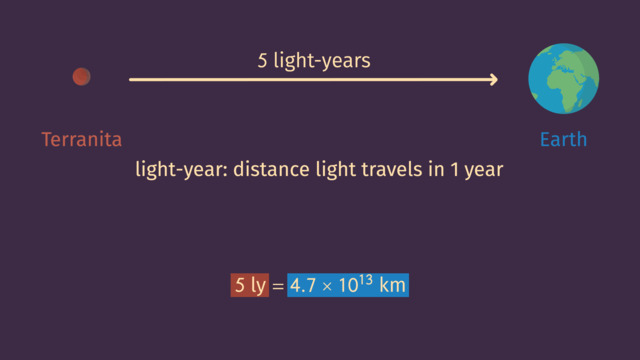# Choice of UnitRating

Be the first to give a rating!
The authorsChris S.

## Basics on the topicChoice of Unit

After this lesson you will be able to determine appropriate units for any measurement, and create new units of measurement, specifically for a situation.

The lesson begins with the criteria you should use to determine which unit is best for any given measurement. This leads to an example where creating a new unit of measurement is necessary.

Learn about choosing units of measurement, by helping Chad on his interplanetary vacation to Earth!

This video includes key concepts, notation, and vocabulary such as: scientific notation (a method of writing numbers in terms of a decimal number between 1 and 10, multiplied by a power of ten); light year (the distance light travels in 1 year); and unit of measurement (a quantity used to express the magnitude of another quantity, such as meters, grams, or degrees).

Before watching this video, you should already be familiar with numbers written in scientific notation and how to convert between units of measurement.

After watching this video, you will be prepared to compare and perform arithmetic operations on numbers written in scientific notation.

Common Core Standard(s) in focus: 8.EE.4 A video intended for math students in the 8th grade Recommended for students who are 13-14 years old